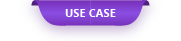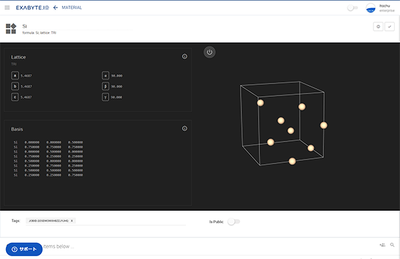First-principles calculation has become an indispensable tool for material design in recent years because it can calculate various physical properties.

# Use Case: Calculation of elastic constant of crystal structure

#### Overview

First-principles calculation has become an indispensable tool for material design in recent years because it can calculate various physical properties. I will introduce one of the physical characteristics, the calculation of the elastic constant of the crystal structure.

#### Modeling

From Exabyte.io, connect to Material Projects of the crystal database and download and use the crystal structure of Si and 6H-SiC.

#### Calculation

Use the workflow function of Exabyte.io to call the calculation flow to obtain the elastic constant and perform the calculation. Finally, after setting the calculation core to be used for the calculation, perform cloud computing.

#### Calculation result

The elastic constants of Si crystals are shown below.

###### Table 1 Elastic modulus of Si crystal (GPa)

Although there are some deviations from the experimental values, the trends are in agreement.

The elastic constants of 6H-SiC crystals are shown below.

###### Table 2 6H-SiC Crystal Elastic Modulus (GPa)

You can see that the calculated value is close to the experimental value. By using first-principles calculations, elastic constants can be predicted.

#### References

 Kamitani, K., M. Grimsditch, JC Nipko, C.-K. Loong, M. Okada, I. Kimura, The elastic constants of silicon carbide: A Brillouin-scattering study of 4H and 6H SiC single crystals. J. Appl. Phys. 82, 6 (1997), 3152-3154.

Original Source from: https://ctc-mi-solution.com/結晶構造の弾性定数の計算/